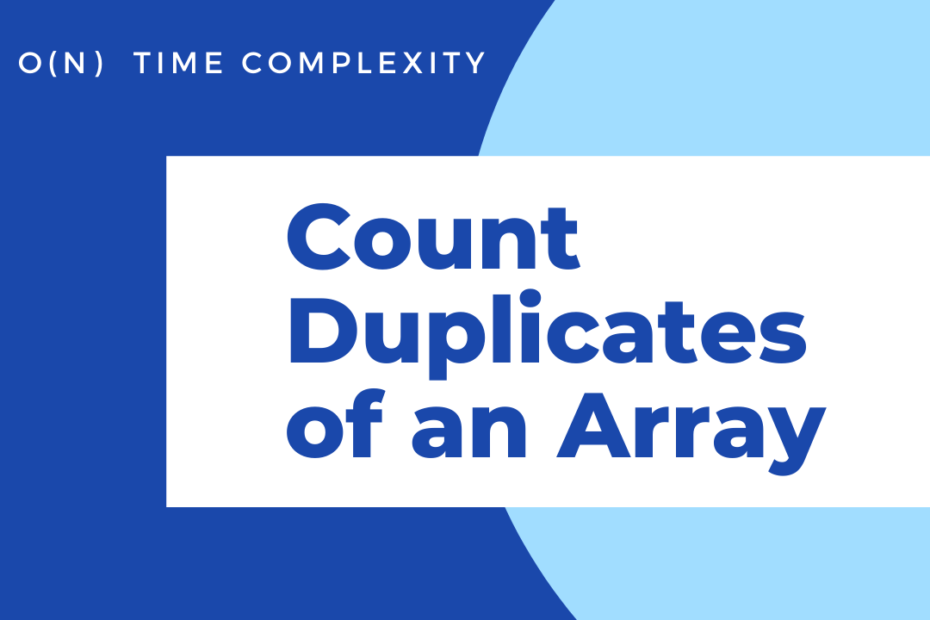# Array## Count the Duplicates of Array O(N)

• DSA

In the given problem, we have a sorted array, and we need to count the duplicates of each element. In simple words, we should print the no.of times each element occurs. Example Solution approach Since we have a sorted array, things become much easier. Once we traverse through an element,… Read More »Count the Duplicates of Array O(N)## Anagram in Strings

• DSA

Given, two strings and we need to check if those strings form an anagram. If you are wondering what’s an Anagram? When the characters of one word are obtained from rearranging the letters/characters of another word, they are called anagrams. Example Note: Important!, Anagrams can be a single word or… Read More »Anagram in Strings## Target Sum – Single Iteration O(N)

• DSA

Given, a sorted array of elements and we need to find all the pairs of elements that add up equally to a given target sum number, this can be done usually done using two loops, like a brute force method where all the elements are compared with every other element… Read More »Target Sum – Single Iteration O(N)## Two Sum LeetCode Optimized

• DSA

The Two Sum problem from LeetCode. Given an array of numbers and a target number and we should return the indices of the two numbers that sum up to the target. Let our target be 7 and then if our array contains 3,4 and 3+4=7 we will return the index… Read More »Two Sum LeetCode Optimized## Squares of a Sorted Array LeetCode O(N)

• DSA

In this problem, given a sorted array in decreasing order and we should return the squares of each number and in the same ascending order, and the one thing we need to take care of is that the negative numbers, which when squared disturb the ascending ordered array. Problem Statement… Read More »Squares of a Sorted Array LeetCode O(N)## Single Number in Array LeetCode C++

• DSA

Program to Find the Single Number in an Array LeetCode problem. In this question, we have an array containing numbers in pairs, but a single number appears only once in an array and we need to print that out. Note: Our solution needs to have a Linear Runtime Complexity and… Read More »Single Number in Array LeetCode C++